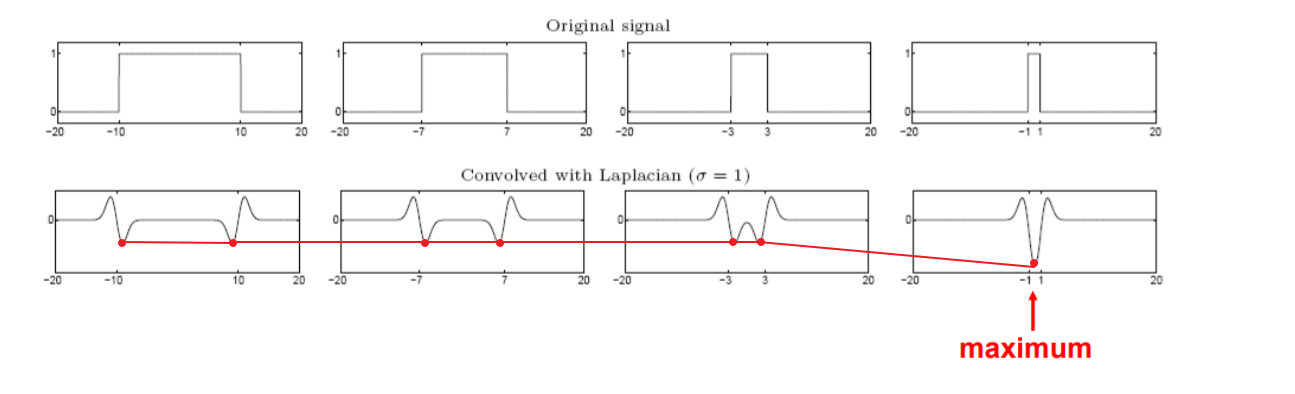# 区域检测——Blob & SIFT“当尺度改变时控制每个圆内的内容不变”

## 二维空间的Blob的检测

$$\nabla^2 g=\frac{\partial^2 g}{\partial x^2}+\frac{\partial^2 g}{\partial y^2}\Longrightarrow \nabla_{norm}^2 g=\sigma^2(\frac{\partial^2 g}{\partial x^2}+\frac{\partial^2 g}{\partial y^2})$$

Laplcain算子中的$\sigma$与检测对象画出的圆的半径$r$的关系

$$\Downarrow$$

$$x^2+y^2-2\sigma^2=0$$ 得到：$r=\sqrt{2}\sigma$

## SIFT特征

DoG模板 DoG的函数图像与Laplacian核很相似，具有相似的性质，但使用的时两个高斯差分来定义，大的高斯核可以使用小的高斯核来计算，大大减少了计算量

$$G(x,y,k\sigma)-G(x,y,\sigma)\approx(k-1)\sigma^2\nabla^2G$$

1. 高斯空间中的模板利用DoG算法直接从前一层的基础上计算，这样就形成一个DoG空间，得到的模板与与高斯空间相差一个常数项$(k-1)$
2. 计算大尺度的模板时不改变参数值，改变图像大小，例如：将图像缩小一倍，不改变模板尺度得到效果和增大模板尺度不改变图像大小的效果相同，计算四倍尺度的值就将图像缩小四倍，$\sqrt{2}\sigma$的尺度在缩小一倍的图像上的对应尺度为$2\sqrt{2}\sigma$
3. $k=2^{1/s}$：$s$表示要输出的尺度有多少个，利用$s$来计算$k$,例如下图是输出尺度为$s=2$时的示例，此时$k=\sqrt{2}$,二倍尺度状态下的起始模板可以由一倍尺度的$k^2\sigma=2\sigma$下采样得到
4. 模板尺度通常取$2$的等比数列$(1,2,4,8,16……)$

SIFT仿射变换

1. 先确定一个圆

2. 将圆内的所有像素拿出来计算$M$矩阵

3. 比较计算出来的$\lambda_1,\lambda_2$

4. 将较小的$\lambda$的方向进行缩小

5. 再将上一步缩小后的区域（椭圆）内的像素拿出来计算$M$矩阵

6. 重复上述步骤，逐步迭代。直至$\lambda_1,\lambda_2$近似相等，说明区域边缘的梯度变化近似一致

7. 将椭圆转换到一样大小的圆中

1. 计算圆内每个像素的梯度强度和方向

2. 将梯度方向量化成八份，给对应的直方图投票，票数就是梯度的大小

3. 统计完之后选择票数最高的方向作为，作为圆内像素整体的梯度方向，将方向转换到$0^\circ$，将整个圆进行相同的旋转

4. 决绝明暗不一致：将圆均分成16格，每个格代表一个区域，统计每个区域的方向量化梯度（两化成八个角度，长度代表梯度大小），每个区域中由一个“8位”向量表示，将16个区域的向量拉直就得到一个 $8\times16=128$ 的向量来描述这个圆内的内容，最后比较每个圆的128个数来判断两个圆内容的相似程度